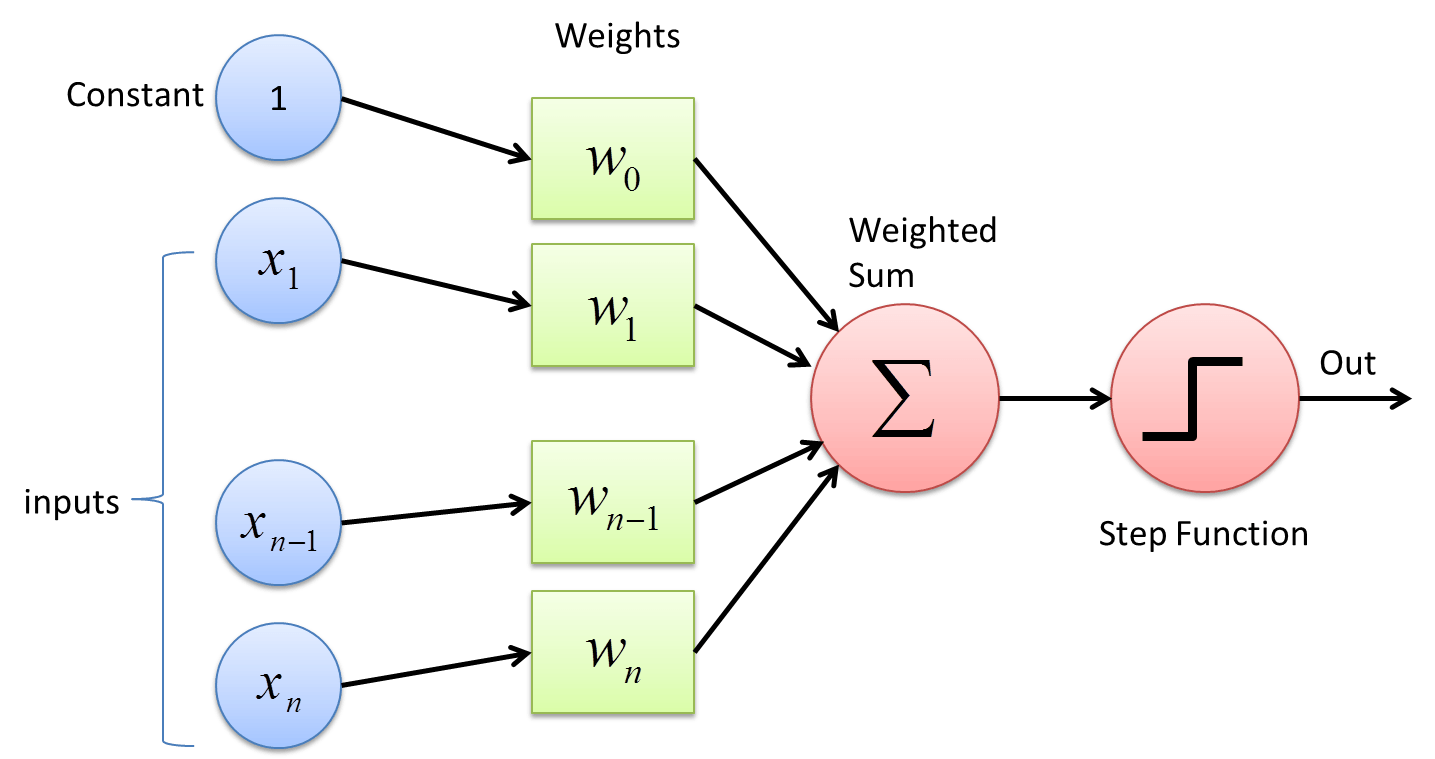# Day 19 Perceptron¶

## Nov. 19, 2020¶# Announcements¶

• Homework 5 Working with Tensorflow. Due 12/4. This is the last homework assignment!
• Projects Rubric posted to D2L.
• Due finals week
• 8-10 minute video presentation + documented notebook on your analysis
• 3 In-class work periods for the project

# Calendar¶

## This week¶

• Thursday: Day 19 Perceptron model

## Thanksgiving week¶

• Tuesday 11/24: Project work day 1
• Thursday 11/26: No class

## Week after Thanksgiving¶

• Tuesday 12/1: Day 20 Neural Networks 1
• Thursday 12/3: Day 21 Neural Networks 2

## Last week of classes¶

• Tuesday 12/8: Project work day 2
• Thursday 12/10: Project work day 3

# Pre-Class¶

• What is the perceptron model doing?
• How do we take the mathematics and make it into code?
• How is this different from a neural network?

# Perceptron (single layer neural network)¶# Neural Network ("multi layer perceptron")¶# How does a Perceptron model classify points?¶

## 2D example¶• A perceptron model is trying to find a line to seperate the classes
• Each point in a 2D space has a location $(x_1, x_2)$; basically feature_1 and feature_2
• A line in that space would have the normal form $A + Bx_1 + Cx_2 = 0$ or $x_2 = -\dfrac{B}{C}x_1 - \dfrac{A}{C}$
• Using an iterative approach, a Perceptron model tries to find $A$, $B$, and $C$.

# One prediction predict()¶

The perceptron model iteratively determines $A$, $B$, and $C$ by looking at every point in the data it is trained on.

• Take the location of one data point plus a constant (the "bias"; e.g., 1) and take the dot product with an initial guess of the weights (e.g., $\vec{w} = (1,1,1)$). $$result = \vec{x} \cdot \vec{w} = (x_1, x_2, 1) \cdot (1,1,1) = x_1 + x_2 + 1$$
• If $x_1 = 2$ and $x_2 = 3$, then the $result = (2+3+1) = 6$

Because this is greater than zero, we predict it to be in class 1

if result > 0: predict class 1 and return 1; else: predict class 2 and return -1

For our example, we return 1!

But we know the class (because we are using supervised learning)!

# Compare to actual class and update weights¶

• Originally we guessed the weights $\vec{w} = (1,1,1)$, we can use the misclassifications to update the weights.

Let's assume we were wrong, so the data is actually in class 2.

That update uses this equation: $$\vec{w}_{new} = \vec{w}_{old} + \eta*d*\vec{x}$$ where $\eta$ is the learning rate and $d$ = actual_class_value - predicted_class_value (as long as classes are 1 and -1)

# Continuing example¶

We predicted class 1 (class_label=1), but the data is in class 2 (class_label=-1). So the update to the weights is:

$$update = \eta*d*\vec{x} = \eta*(-1 - (1))*(2,3,1)$$$$update = \eta*(-2)*(2,3,1) = (-4,-6,-2)*\eta$$

where we choose $\eta$, let's take it to be 0.01. So the update is:

$$update = (-4,-6,-2)*0.01 = (-0.04, -0.06, -0.02)$$

We add this to the guessed weights:

$$\vec{w}_{new} = \vec{w}_{old} + update = (1,1,1) + (-0.04, -0.06, -0.02) = (0.96,0.94,0.98)$$

# What is we guess correctly?¶

In that case, the predicted and known classes are the same, so the update is:

$$update = \eta*d*\vec{x} = \eta*(-1 - (-1))*(2,3,1)$$$$update = \eta*(0)*(2,3,1) = (-4,-6,-2)*\eta = 0$$

And there's no change to the weights because we did ok!

This means perceptrons don't find the "best line" just a line that separates the data.

# So how do we fit the model? fit()¶

for the number of iterations we choose: for the data we have: predict the class update the weights